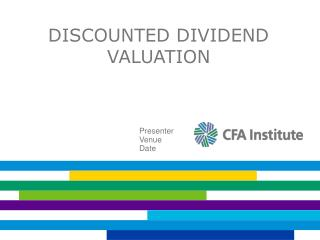DownloadDownload PresentationDiscounted Dividend Valuation

# Discounted Dividend Valuation

Download Presentation## Discounted Dividend Valuation

- - - - - - - - - - - - - - - - - - - - - - - - - - - E N D - - - - - - - - - - - - - - - - - - - - - - - - - - -
##### Presentation Transcript

1. Discounted Dividend Valuation Presenter Venue Date

2. Discounted Cash Flow Models

3. Choice of Discounted Cash Flow Models

4. Valuing Common Stock Using a Multi-period DDM

5. Example: Valuing Common Stock Using a Multperiod DDM

6. Example: Valuing Common Stock using a Multperiod DDM

7. Valuing Common Stock Using the Gordon Growth Model

8. Example: Valuing Common Stock Using the Gordon Growth Model

9. Valuing Common Stock Using the Gordon Growth Model

10. Example: Valuing Preferred Stock

11. Example: Calculating the Implied Growth Rate Using the Gordon Growth model Using the previous common stock example and the current stock price of \$24, what is the implied growth rate?

12. Example: Calculating the Implied Required Return Using the Gordon Growth Model Using the previous common stock example and the current stock price of \$24, what is the implied required return?

13. Present Value of Growth Opportunities

14. Present Value of Growth Opportunities

15. Example: Present Value of Growth Opportunities

16. Example: Present Value of Growth Opportunities

17. Example: Present Value of Growth Opportunities

18. Issues Using the Gordon Growth Model

19. Choice of Discounted Cash Flow Models

20. General Two-Stage DDM

21. Example: General Two-Stage DDM Current dividend = \$2.00 • Growth Current dividend = \$2.00 • Growth for next three years = 15 percent • Long-term growth = 4 percent • Required return = 10 percent for next three years = 15 percent Long-term growth = 4 percent Required return = 10 percent

22. Example: General Two-Stage DDM • Step 1: Calculate the first three dividends: • D1 = \$2.00 x (1.15) = \$2.30 • D2 = \$2.30 x (1.15) = \$2.6450 • D3 = \$2.6450 x (1.15) = \$3.0418 • Step 2: Calculate the year 4 dividend: • D4 = \$3.0418 x (1.04) = \$3.1634 • Step 3: Calculate the value of the constant growth dividends: • V3 = \$3.1634 / (0.10 – 0.04) = \$52.7237

23. Example: General Two-Stage DDM

24. Example: General Two-Stage DDM • Using the previous example, now we’ll use the trailing P/E to determine the terminal value • The D4 is \$3.1634 • Assume also that the projected P/E is 13.0 in year 4 and that the firm will pay out 60 percent of earnings as dividends • Year 4 earnings are then \$3.1634 / 0.60 = \$5.2724 • The stock price in year 4 is then \$5.2724 × 13 = \$68.54

25. Example: General Two-Stage DDM

26. Two-Stage H-Model

27. Example: Two-Stage H-Model

28. Example: Two-Stage H-Model

29. Solving for the Required Return Using the Two-Stage H-Model

30. Example: Three-Stage Model • Firm pays a current dividend of \$1.00 • Growth rate is 20 percent for next two years • Growth then declines over six years to stable rate of 5 percent • Required return is 10 percent • Current stock price is \$50

31. Three-Stage Model Assumes three distinct growth stages: • First stage of growth • Second stage of growth • Stable-growth phase H-model can be used for last two stages if growth declines linearly

32. THREE-STAGE MODEL EXAMPLE

33. Estimating the Growth Rate

34. The Sustainable Growth Rate

35. The DuPont Model

36. Example: DuPont Model

37. Example: DuPont Model

38. Summary

39. Summary

40. Summary